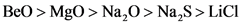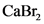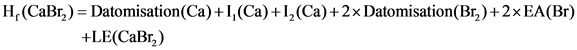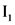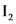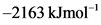# Rank the following ionic compounds by lattice energy. rank from highest to lowest lattice energy.

a) Rank the following ionic compounds by the magnitude of their
lattice energy. Rank from highest to lowest magnitude of lattice
energy. Highest magnitude to Lowest Magnitude LiCl, MgO, Na2O, BeO,
Na2s b) Given the following thermodynamic data, calculate the
lattice energy of CaBr2(s). Term Value (kJ/mol) ΔH∘f[CaBr2(s)] -675
ΔH∘f[Ca(g)] 179 ΔH∘f[Br(g)] 112 I1(Ca) 590. I2(Ca) 1145 E(Br) -325
Express your answer as an integer, and include the appropriate
units.

General guidance

Concepts and reason
The problem is based on the concept of lattice energy. Lattice energy is defined as the amount of energy released when gaseous ions combine to form ionic solids.

Fundamentals

Lattice energy is the energy released when a crystal lattice is formed. It can also be defined as the energy required to break the crystal lattice into gaseous ions. Usually, it is the energy released so it is taken as negative. Lattice energy depends on the distance between the ions and charge on the ions. As the ionic charge increases, the lattice energy also increases and becomes more negative. And as the ions get closer, then also there is an increase in lattice energy.

Step-by-step

Step 1 of 2

(A)
Lattice energy depends on the distance between ions and charge on ions.
Depending on this condition the order is as follows:

Part A
The decreasing order of the ionic compounds by the magnitude of their lattice energies is as follows:

Since Beryllium has the smallest ionic radius amongst the given ionic compounds, its lattice energy is the highest. Lithium ion and chloride ion carry the lowest charge; therefore, the lattice energy of lithium chloride is the lowest.

Though the size of Li is greater than Be but it carries lesser charge than Be. Therefore, it has lower lattice energy than Be.

The Lattice energy of is calculated with the help of the following formula.

Step 2 of 2

(2)
Calculate the lattice energy as follows:

Here, and represent the ionization energy, EA represents the electron affinity, LE represents lattice energy.
Substituting the values in the above equation:

Therefore, the lattice energy of is .

Part 2
The lattice energy of is .

Enthalpy of formation is the sum of all the enthalpies that includes enthalpy of atomization, electron affinity and lattice energy.

Whenever energy is released it is take as negative and energy required is taken as positive.
Do not interchange the signs.

Part A
The decreasing order of the ionic compounds by the magnitude of their lattice energies is as follows:

Part 2
The lattice energy of is .

Part A
The decreasing order of the ionic compounds by the magnitude of their lattice energies is as follows:

Part 2
The lattice energy of is .

BeO > MgO > Na,O > Na S> Lici
CaBr
H,(CaBr) = Datomisation(Ca)+1, (Ca)+1,(Ca)+2x Datomisation(Bry)+2xEA(Br) +LE(CaBr)
H,(CaBr) = Datomisation(Ca)+1, (Ca)+1,(Ca)+2x Datomisation(Bry)+2xEA(Br) +LE(CaBr)
-675 kJmol”‘ =179 kJmol-‘ +590 kJmol-‘ +1145 kJmol”‘ + (2×112) kJmol’ +(2x-325) kJmol-‘ +LE(CaBr) LE(CaBr) = -675 kJmol-‘-179 kJmol”‘ – 590 kJmol”‘-1145 kJmol -224 kJmol-‘ +650 kJmol-‘ = -2163 kJmol-‘
CaBr
-2163 kJmol
BeO > MgO > Na,O > Na S> Lici
BeO > MgO > Na,O > Na S> Lici
Be0
CaBr
-2163 kJmol
CaBr
We were unable to transcribe this image